Simulation of the 3D viscoelastic free surface flow by a parallel corrected particle scheme
Department of Mathematics, Yangzhou University, Yangzhou 225002, China

† Corresponding author. E-mail: rjl20081223@126.com

Project supported by the Natural Science Foundation of Jiangsu Province, China (Grant Nos. BK20130436 and BK20150436) and the Natural Science Foundation of the Higher Education Institutions of Jiangsu Province, China (Grant No. 15KJB110025).

Abstract
Abstract

In this work, the behavior of the three-dimensional (3D) jet coiling based on the viscoelastic Oldroyd-B model is investigated by a corrected particle scheme, which is named the smoothed particle hydrodynamics with corrected symmetric kernel gradient and shifting particle technique (SPH_CS_SP) method. The accuracy and stability of SPH_CS_SP method is first tested by solving Poiseuille flow and Taylor–Green flow. Then the capacity for the SPH_CS_SP method to solve the viscoelastic fluid is verified by the polymer flow through a periodic array of cylinders. Moreover, the convergence of the SPH_CS_SP method is also investigated. Finally, the proposed method is further applied to the 3D viscoelastic jet coiling problem, and the influences of macroscopic parameters on the jet coiling are discussed. The numerical results show that the SPH_CS_SP method has higher accuracy and better stability than the traditional SPH method and other corrected SPH method, and can improve the tensile instability.

1. Introduction

The complex jet coiling phenomena, although they play an important role in many industrial processes, such as extrusion, and container filling with polymers, are still less well understood in terms of fundamental fluid dynamics. In order to predict the complex rheological phenomena in a real three-dimensional (3D) viscoelastic jet buckling process, a number of grid-based numerical methods have been proposed, such as the finite difference method (FDM), finite element method (FEM), finite volume method (FVM), etc. The mesh-based methods mentioned above have been applied to many fluid mechanic fields.

However, these grid-based methods also suffer numerical difficulties, such as severe mesh distortion, and the expensive cost in the mesh generation process. As one may expect, the various mesh-free methods in a Lagrangian framework have been proposed to solve the fluid dynamic problems, such as the smoothed particle hydrodynamics (SPH) method,[4,5] element-free Galerkin (EFG) method, reproducing kernel particle method (RKPM), and so on.

The SPH method is a pure meshless method which employs Lagrangian description of motion. Recently, the SPH method has been extensively applied to the fluid dynamic areas due to its merits, such as viscous flows, free surface flows,[5,10,11] incompressible fluids,[12,13] multi-phase flows, and non-Newtonian fluid flows.[13,15] However, the application of the SPH method to the viscoelastic fluid mainly focuses on the two-dimensional (2D) viscoelastic jet buckling, and the application of the SPH method to the 3D viscoelastic jet coiling has received a little attention recently. The reason is that the SPH method usually suffers two major drawbacks, that is, the particle inconsistency[16,17] for uninformed particle distributions/near the boundary domain and the numerical/tensile instability. In order to restore the consistency of the kernel and gradient particle approximations of SPH, some improved SPH methods based on the Taylor series expansion have been proposed, such as the corrected smoothed particle hydrodynamics method (CSPM), finite particle method (FPM),[11,20] the modified SPH (MSPH) method, and the symmetric SPH (SSPH) method. Although these improved methods have higher accuracy than the traditional SPH (TSPH) method, some disadvantages still exist and make it more difficult to be extended to the complex fluid dynamic problems, the choice of the kernel function is limited for solving the partial differential equations (PDEs) with higher derivatives; the local matrix is asymmetric, which can cause numerical instability; its computational efficiency is lower than that of TSPH, such as the MSPH method and SSPH method. Therefore, a corrected kernel gradient scheme has been introduced into the TSPH method and extensively applied to Newtonian fluid[24,25] in recent years. However, the corrected kernel gradient scheme has been rarely extended to simulate the viscoelastic jet buckling, especially the 3D simulations of the viscoelastic jet coiling.

In the present paper, the 3D viscoelastic jet coiling process based on the Oldroyd-B model is simulated and analyzed by a parallel coupled corrected particle scheme named the SPH_CS_SP (SPH with a corrected symmetric kernel gradient scheme and shifting particle technique) method. Some special phenomena of the 3D jet coiling are observed and the physical explanations of these complex phenomena are also analyzed. The proposed particle method combines three improved concepts, that is, introducing the incompressible condition in the momentum equation to achieve better robustness, extending the corrected symmetric kernel gradient scheme to further improve the numerical accuracy and employing the shifting particle technique to overcome the tensile instability. It should be noted that the main difference between the proposed SPH_CS_SP method and other improved SPH methods lies in the following respects: (i) the obtained discretized form of momentum equation is different from those in Refs.  and ; (ii) the shifting particle technique is first applied to the viscoelastic fluid flows; (iii) the presented corrected SPH method is different from the particle methods in Refs.  and , which have not been extended to simulate the 3D polymer free surface flow.

The rest of this paper is organized as follows. The governing equations and the viscoelastic constitutive model are introduced in Section 2. In Section 3, the details of the proposed SPH_CS_SP scheme for the viscoelastic fluid are described. The accuracy and robustness of SPH_CS are tested by simulating the Poiseuille flow in Section 4. Moreover, the necessity of enforcing the shifting particles technique is also investigated by simulating the Taylor–Green flow in Section 4. In Section 5, the capacity for SPH_CS_SP to simulate the viscoelastic flow is verified by the polymer flow around a periodic array of cylinders. In Section 6, the proposed particle method with MPI parallelization technique is extended to simulate the 3D viscoelastic jet coiling problem in the filling process. Some conclusions are drawn from the present study in Section 7.

2. Governing equations based on the Oldroyd-B model

In the 3D Lagrangian frame, the governing equations of a weakly compressible fluid can be described as follows: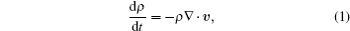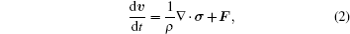where υ = (u,v,w) is the velocity vector, ρ is the fluid density, t is the time, F is the body force, and d/dt is the material derivative d/dt = /∂t + υ ·∇. The Cauchy stress tensor σ in Eq. (2) is decomposed into the ordinary isotropic pressure p and extra stress tensor To: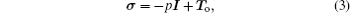where I refers to the identity matrix.

In this paper, we choose the Oldroyd-B model as the constitutive equation, which represents the basic viscoelastic fluid and shows complex rheological behavior of polymer flow. The extra stress tensor To for the Oldroyd-B model is usually decomposed into viscous 2ηsD and polymeric contributions τ: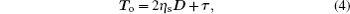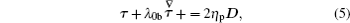where the rate of deformation tensor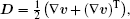λ0b is the relaxation time, and the symbol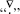represents the following upper-convected derivative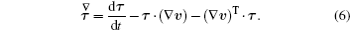Moreover, some parameters are introduced, that is, the total viscosity η = ηs + ηp, the ratio of Newtonian and polymeric viscosity β0 = ηs/(ηs + ηp), the Reynolds number Re = (ρUL)/η, and the Weissenberg number We = (λ0bU)/L, where U and L represent the characteristic velocity and length, respectively.

Like many previous studies,[4,9] here we also treat the incompressible flows as a slightly compressible fluid by a suitable equation of state, that is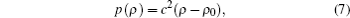where c is the speed of sound, and ρ0 is the reference density. It can be shown that the density variation is proportional to the Mach number M (MV/c, where V is a typical reference velocity).[4,9]

3. SPH_CS_SP method for viscoelastic fluid

In order to accurately simulate the viscoelastic fluid flow, a new corrected particle scheme is proposed. It mainly combines three different improvements, that is, introducing the incompressible condition in the momentum equation to achieve better robustness, applying the corrected symmetric kernel gradient method to the discrete form of the first derivative to improve the numerical accuracy, and employing the shifting particle technique to overcome the tensile instability.[25,26,28]

As is well known, in the traditional SPH method, the particle approximation for a function f and its first derivative at particle i can be written as[4,5]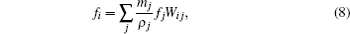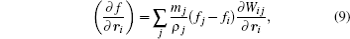where mj and ρj are the mass and the density of the neighbor particle j located in the support domain of particle i, respectively, W is the kernel function which usually satisfies some properties, Wij = W (|rirj|,h), ∂Wij/ri = ∂W(|rirj|,h)/ri, h being the smoothing length.

The kernel function is related not only to the accuracy but also to the efficiency and the stability of the resulting algorithm. As shown in Ref. , the Wendland function can produce more accurate results than the common spline kernel functions. So the following quintic Wendland kernel function is adopted: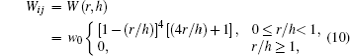where the r = |rirj| and the normalization factor w0 is 7/(πh2.)

According to Eq. (9), the discrete scheme of the continuity equation (1) is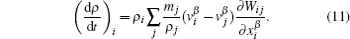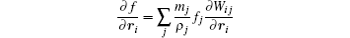and the following identity: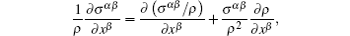we can obtain the conventional discretized scheme of the momentum equation (2), which is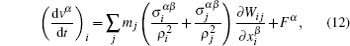where vβ is the β-th component of the fluid velocity, σα β is the (α,β)-th component of the total stress tensor, and xβ is the component of spatial coordinate.

The particle discretized scheme of total stress equation (3) can be defined as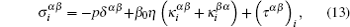where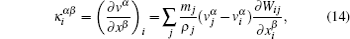and the discretized scheme of the constitutive equation (5) is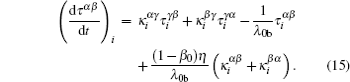3.2. SPH_CS_SP method

The TSPH discretized formulas (11)–(15) for the viscoelastic Oldroyd-B fluid are directly derived from the TSPH discrete formula for the viscous fluid. As is well known, the TSPH formula for the viscous flow suffers low numerical accuracy and instability, especially for the non-uniform distributed particles and boundary particles (see Refs. , , and ). Moreover, the influence of elastic stress on the numerical simulations of viscoelastic fluid flow is also significant, which easily leads to the instability of numerical schemes for the viscoelastic flow. Therefore, in order to obtain a particle method that possesses higher accuracy and better stability, we introduce three improvements[27,28] into the TSPH formulas (11)–(15). The improvements are described in detail as follows.

Firstly, introducing the incompressible condition into momentum equation (2) leads to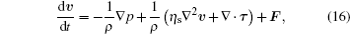and considering the discrete scheme of the Laplace operator, the following discretized scheme of the momentum equation (16) is obtained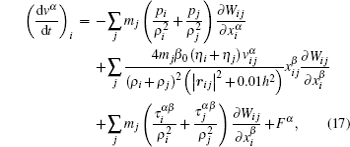where rij = rirj.

Secondly, the corrected symmetric kernel gradient scheme and the Taylor expansion concept are also applied to the first-order derivative and the elastic stress part of Eq. (12) to restore the particle approximation and consistency and improve the numerical accuracy and stability of TSPH method, respectively. Thus the following corrected symmetric SPH (SPH_CS) scheme for the viscoelastic Oldroyd-B fluid is obtained: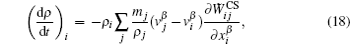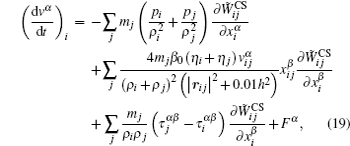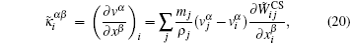where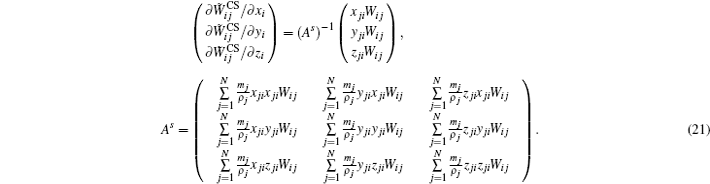It should be noted that the discretized form Eq. (19) for the momentum equation is different from that in Ref. , especially the corrected discretized form of polymer elastic stress in accordance with the Taylor series concept in Eq. (19). Also, the local matrix equation (21) is different from those in Refs.  and .

At the end of each time-step, the position of each particle is updated by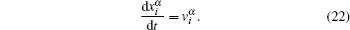Finally, a shifting particle technique, which is applied only to the viscous fluid now, is extended to the viscoelastic fluid to obtain a uniform particle distribution and further improve the tensile instability. Next, we describe the shifting particle technique in detail.

After each time-step, the position of particle i is shifted according to the following formula: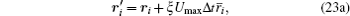where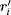is the particle position after shifting, ξ is a constant that ranges from 0.01 to 0.1, Umax is the maximum particle velocity or the characteristic velocity of flow, Δt is the time-step, and the shifting vector is defined as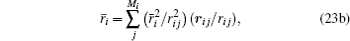where Mi is the number of neighboring particles of the particle i, rij = |rij|, and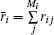is the average particle spacing in the neighborhood of i. The shifting vector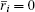when the fluid particles near the particle i are uniformly distributed.

For simplicity, the method that combines the three different improvements is named the SPH_CS_SP method.

3.3. Boundary treatment

In the SPH simulations, the boundary treatment is an important problem. Next, we will describe the boundary treatment used in this paper.

For the solid boundary, the approach of two types of virtual particles is extended to simulating the viscoelastic fluid flows in this paper. The first type of particle is placed on the solid wall, namely “wall particle”. The pressure and the components of the velocity of the wall particle i are calculated according to the following formula: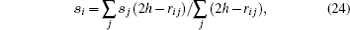where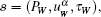j represents the indices of the fluid particles within the influence domain of the boundary particle i.

The second type of particle, called “dummy particle”, is placed just outside the solid wall, in a way which is similar to how the wall particle is located. In general, three layers of dummy particles are chosen. The pressure, velocity and extra stress tensor on the dummy particles are computed by the following formula: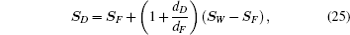where S represents the vector of variables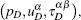dD and dF are the normal distances of the dummy particles D and the fluid particle F to solid wall, respectively. To specify the values of SF, the interpolation formula (24) is applied again. For the periodic boundary, we adopt an established molecular dynamics routine with SPH particles crossing the boundary being re-inserted on the opposite side of the simulation box.

3.4. Time integration scheme

In order to solve the system of ordinary differential equations (15), (18), (19), and (22), we choose the predictor–corrector scheme, which has second-order accuracy and better stability. The predictor step consists of a Eulerian explicit evaluation of all quantities for each particle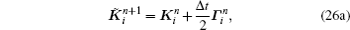where Ki represents the vector of the unknown variables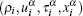and Γi denotes the vector of the right-hand side of each of Eqs. (15), (18), (19), and (22). In the corrected step, the updated value of at the end of each time step is given by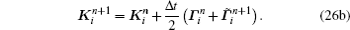As is well known, the time-step and space-step must satisfy the well-known Courant–Friedrichs–Lewy (CFL) condition for ensuring the numerical stability. According to Refs.  and , we may choose the following stability condition: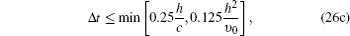where υ0 = η/ρ0 is the kinematic viscosity.

4. Valid test for the SPH_CS_SP method
4.1. Accuracy and the stability of SPH_CS_CP method

In this section, the Poiseuille flow of Oldroyd-B fluid is simulated by SPH_CS_CP method to test the capacity for the proposed SPH_CS method to solve the transient viscoelastic fluid, and the numerical results are compared with those obtained by TSPH method and the analytical solutions. It should be noted that the tensile instability does not exist in the simulations of the Poiseuille flow, and the shifting particle technique is not considered in this subsection.

Figure 1 shows the velocity u and the stress τxy profiles at different times for the Poiseuille flow of Oldroyd-B fluid. Here the uniformed particle distribution is used. The employed non-dimensional parameters are as follows: two parallels are located at y = 0 and y = 1, the density ρ0 = 1, the characteristic velocity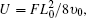the ratio of viscosity β0 = 0.3, the kinematic viscosity υ0 = 2, the body force F = 1, Re ≈ 0.03, the relaxation time, λob = 0.2, 4 which correspond to We = 0.012, 0.25, respectively, the sound speed c = 10Umax, the time-step Δt = 10−4, the smoothing length h = 2.5d0 (d0 is the initial distance between two particles), the number of fluid particles is 31 × 61.

 Figure Option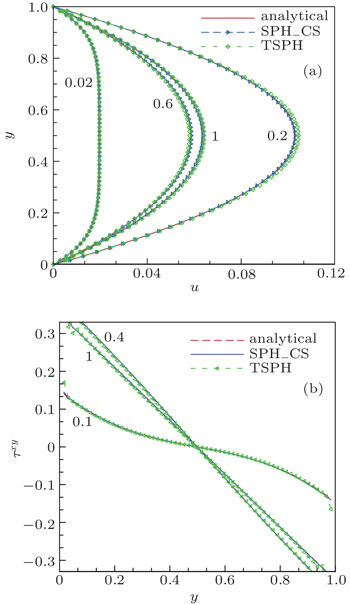Fig. 1. Results for the Oldroyd-B fluid at different times with Re ≈ 0.03: (a) u(y); (b) τxy(y).

Table 1 gives the relative L1-error norms of the velocity profiles in Fig. 1(a). The relative L1-error norm is defined as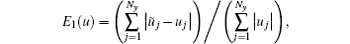where Ny denotes the particle number along the y axis, j and uj are the numerical and the analytical solution at position xj, respectively.

From Fig. 1 and Table 1, we can observe that the SPH_CS results are much closer to the analytical solutions than the TSPH results, and the SPH_CS method has faster numerical convergence than the TSPH method for solving the viscoelastic fluid flow under the uniformed particle distributions. It is worth noting that the shear elastic stress obtained by the TSPH method has small oscillation near the boundary, which leads to the numerical instability varying with time. Meanwhile, the overshooting phenomenon can be observed in the Oldroyd-B fluid flow.Table 1.

Relative L1-error norm of the velocity profiles for the case of Fig. 1(a).

.

Figure 2 shows the velocity profiles for the Poiseuille flow of Oldroyd-B fluid with different values of We and Re. In Fig. 2(a), the parameters are the same as those in Fig. 1. In Fig. 2(b), the parameters are the same as those in Fig. 1 except that υ0 = 0.25, F = 0.5, Re = 1, λob = 0.04, and We = 0.01. From Fig. 2, it can be observed that the velocity overshooting behavior becomes more evident and the accuracy of SPH_CS results becomes higher than that of TSPH results with increasing Weissenberg number (Fig. 2(a)). With increasing Reynolds number, the TSPH solutions are much further from the analytical ones with time going by and they appear unstable near boundary domain about t = 0.6 (see Fig. 2(b)).

 Figure Option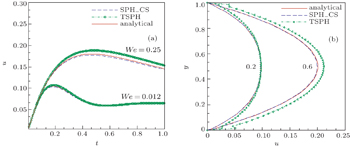Fig. 2. Velocity profiles for the Poiseuille flow of Oldroyd-B fluid with different values of We and Re: (a) Re ≈ 0.03, u(y = 0.5); (b) Re = 1, u(y).

The numerical results in Figs. 1 and 2 show that the SPH_CS has higher accuracy and better robustness than the TSPH for simulating the viscoelastic fluid flow, especially with the larger Weissenberg number or Reynolds number, and the overshooting phenomenon of velocity obtained by the SPH_CS method is more accurate than that obtained by the TSPH method, especially for the larger Weissenberg number.

4.2. Necessity of the shifting particle technique

In this subsection, the 2D Taylor–Green flow of the Newtonian fluid is simulated to show the necessity of the shifting particle technique.

The Taylor–Green flow is a periodic array of decaying vortices in the plane, and its velocity components are given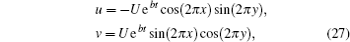where the velocity scale U = 1 m/s, the computational periodic domain [0,1] × [0,1], the kinematic viscosity υ0 = 0.01 m2/s, Reynolds number Re = UL/υ0 = 100, and the decay rate of the velocity field b = −8π2/Re.

Figure 3 shows the particle distributions for the Taylor–Green flow obtained by different numerical methods. It can be observed that the tensile instability in the TSPH results is more serious than that in other numerical results. The quintic Wendland kernel function has better stability than the conventional cubic spline kernel (Figs. 3(a) and 3(b)). The particle distributions obtained by SPH_CS_SP method are more uniform than those obtained by the TSPH method and SPH_CS method due to the effect of shifting particle technique combining with the corrected symmetric kernel gradient scheme and the quintic Wendland kernel function. Moreover, the tensile instability is much improved by the SPH_CS_SP method (Fig. 3(d)).

 Figure Option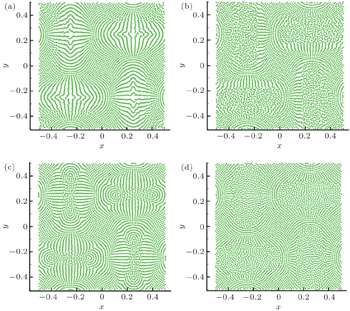Fig. 3. Particle distributions obtained using different numerical methods with Re = 100, t = 0.2 s: (a) TSPH with cubic spline kernel; (b) TSPH with quintic Wendland kernel; (c) SPH_CS with quintic Wendland kernel; (d) SPH_CS_SP with quintic Wendland kernel.

Figure 4 shows the velocity profiles at x/L = 0.0 with Re = 100, t = 0.2 s. The SPH_CS_SP results of velocity component u(y) are much closer to the analytical solutions than the other numerical results, and small oscillations appear in the TSPH results.

 Figure Option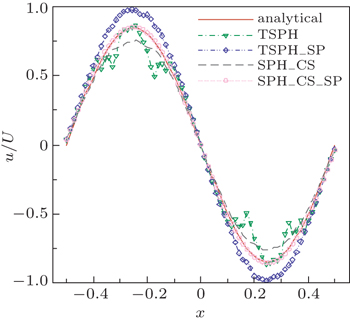Fig. 4. Velocity profiles at x/L = 0.0 m with Re = 100, t = 0.2 s

The numerical results show that the proposed SPH_CS_SP method indeed has higher accuracy and better stability than the TSPH, TSPH_SP and SPH_CS method, and it is necessary to combine the shifting particles technique with the corrected symmetric kernel gradient scheme.

5. The reliability and the convergence of the SPH_CS_SP method

The polymer fluid flow around a periodic array of cylinders is usually regarded as a challenging test for the particle method. Here, we use it to test the reliability of the proposed SPH_CS_SP method to simulate the viscoelastic fluid.

Figure 5 shows the geometric configuration of flow past a periodic array of cylinders and the analog configuration of single cylinder within a periodic lattice. The relevant parameters are as follows: F is an effective body force, rc is the cylinder radius, LH is the half height of two parallel channels, Lc is the distance between two neighbor cylinders, and xc is the distance from the positive direction of x axis to the center of reference cylinder (see Fig. 5(b)). Some non-dimensional parameters are introduced: Ro=xc/rc, Lr = Lc/rc, Re = (ρUrc)/η and We = (λobU)/rc, U′ = u/10km, ταβ = τ αβ/10km, P′ = p/10kn (where km and kn are the integers of scientific computing, km = 4, kn = 3 in these simulations), the first normal stress difference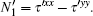Figure Option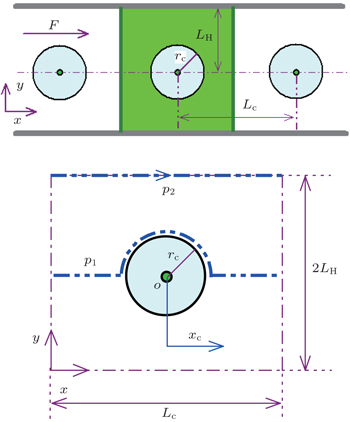Fig. 5. Geometric configuration of flow past a periodic array of cylinders: (a) periodic array of cylinders; (b) analog configuration of a single cylinder.
5.1. Reliability test of numerical simulations

In order to test the reliability of the SPH_CS_SP method of simulating the flow past cylinders, the same example of Newtonian fluid as that in Ref.  is first simulated by the SPH_CS_SP method. All the physical parameters are the same as those in Ref. , i.e., LH = 0.05 m, rc = 0.02 m, F = 5 × 10−5 m·s−2, ρ = 103 kg·m−3, η = 0.1 Pa·s, U = 1.5 × 10−4 m·s−1, c = 0.01 m·s−1, and Re = 0.03; the fluid particles are uniformly distributed in the whole domain, and the particle number Ny = 81 along the y axis. The time step Δt = 5 × 10−3 s, smoothing length h = 2.7d0.

Figure 6 shows the curves of velocity U and the pressure at stable state along different paths (see Fig. 5(b)). It is worth noting that the fluid domain is not confined in Fig. 6, and the Newman boundary condition is enforced at the up and down boundary of the fluid domain. The SPH_CS_SP results are much closer to the FEM results than the TSPH and SPH results in Ref. , the pressure oscillation is more serious for the TSPH and SPH results in Ref.  than for the SPH_CS_CS results, which implies that the pressure oscillation can be well controlled by the SPH_CS_SP method.

 Figure Option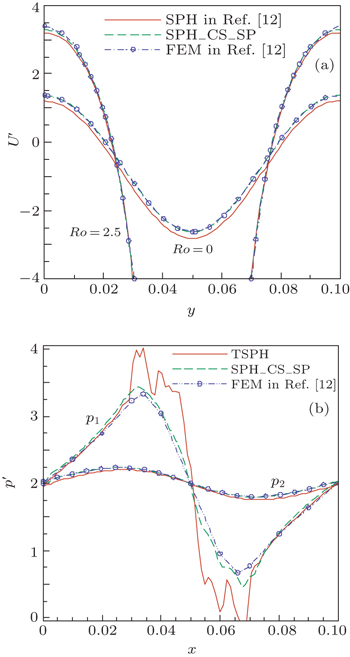Fig. 6. Numerical results for the flow at stable state along different paths: (a) U; (b) pressure.

Figure 7 shows the contours of velocity U and pressure obtained by the SPH_CS_SP method for the flow at the stable state under different boundary conditions (i.e., the flow is confined or not confined in a channel which corresponds to the Newman boundary condition or non-slip wall boundary condition, respectively). Comparing Fig. 7 with Fig. 12, Fig. 14 in Ref.  and Fig. 4 in Ref. , we can find that the numerical solutions obtained by the SPH_CS_SP method are always accurate and reliable.

 Figure Option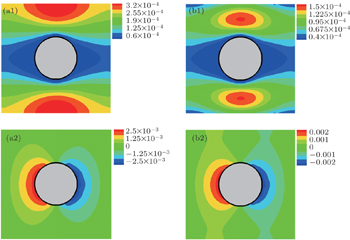Fig. 7. Contour distributions of velocity U [(a1) and (b1)] and pressure [(a2) and (b2)] with different boundary conditions: (a1) and (a2) without confining a channel; (b1) and (b2) confined in a channel.

In order to demonstrate the capacity of the SPH_CS_SP approach for simulating the viscoelastic flow past a periodic array of cylinders, the Oldroyd-B fluid flow past a periodic array of cylinders is simulated and the particle distribution at stable state is shown in Fig. 8. The employed physical quantities are as follows: Lr = 6, LH = 0.04 m, U = 1.2 × 10−4 m·s−1, c = 0.02 m·s−1, Re = 0.024, We = 0.9. Ny = 71, and h = 2.7d0. The other parameters are the same as those in Fig. 6.

Moreover, the following drag for the viscoelastic fluid flow past a cylinder is introduced, which is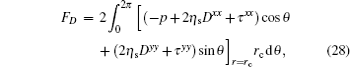and the drag coefficient CD = FD/(ηU).

It can be seen that the so-called tensile instability appears near the cylinder in the SPH results (Fig. 8(a)), and can be improved by the SPH_CS_SP method (Fig. 8(b)).

 Figure Option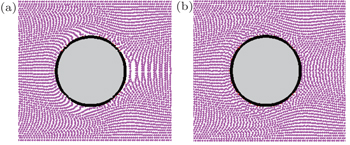Fig. 8. Particle distributions at stable state for Oldroyd-B fluid flow past a periodic array of cylinders with Lr = 6, We = 0.9: (a) SPH in Ref. ; (b) SPH_CS_SP.

From Figs. 68, we can conclude that the SPH_CS_SP method can credibly simulate the polymer flow around a periodic array of cylinders, and has higher accuracy and better stability than the TSPH method or SPH methods in Refs.  and .

5.2. Numerical convergence analysis and drag coefficient calculation

According to Ref. , the numerical convergence of SPH scheme not only depends on the particle numbers, but also relies on the smoothing length. Next, the simple numerical convergence is investigated by increasing the particle number Ny with different values of smoothing length h.

Figure 9 shows the curves of velocity, shear elastic stress and first normal stress difference along different paths with increasing the particle number Ny at Lr = 6, We = 0.06, and Lxy represents the path over the cylinder centerline with the path being at 45° with respect to the horizontal line. It can be found that U, τxy, and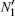have good consistency with the increasing particle numbers, showing that the SPH_CS_SP method is convergent.

 Figure Option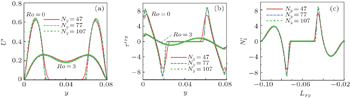Fig. 9. Curves of velocity (a), shear elastic stress (b), and first normal stress difference (c) along different paths with increasing the particle number at Lr = 6 and We = 0.06.

Figure 10 shows the shear elastic stresses along the path Ro = 2 with different values of smoothing length h = 2.0d0, 2.4d0, 2.8d0, Lr = 6, We = 0.8, β0 = 0.2. It can be found that the numerical results have good convergences at h ≥ 2.0d0 in our numerical simulations. Meanwhile, the tensile instability at h = 2.4d0 is more serious than that at h = 2.8d0. The analysis has shown good convergence for the proposed method at h ≥ 2.4d0.

 Figure Option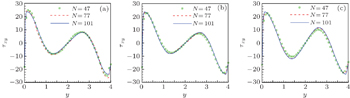Fig. 10. Shear elastic stresses along the path Ro = 2 with different values of smoothing length h (Lr = 6, We = 0.8, β0 = 0.2): (a) h = 2.0d0; (b) h = 2.4d0; (c) h = 2.8d0.

Figures 11(a) and 11(b) show the variations of drag coefficient with the increasing particle number and Weissenberg number respectively. From Fig. 11(a), it can be seen that the drag coefficient for the Newtonian or Oldroyd-B fluid at Lr = 6 reaches a stable value with increasing Ny. The values of drag coefficient for the Newtonian fluid are nicely consistent with those in Refs.  and , the values of drag coefficient for the Oldroyd-B fluid are smaller than those for the Newtonian fluid. The change of the drag coefficient with Lr = 6 is more complex than that with Lr = 2.5 with increasing Weissenberg number (Fig. 11(b)), which is in agreement with the conclusion in Ref. . In a word, the proposed SPH_CS_SP approach has desirable numerical convergence for simulating the problem of flow past a cylinder at low Reynolds number.

 Figure Option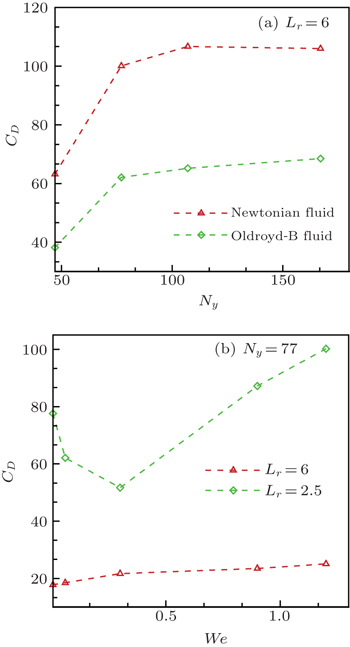Fig. 11. Variations of drag coefficient with (a) Ny and (b) We.
6. Application of the SPH_CS_SP method to 3D viscoelastic free surface flows

In order to demonstrate the ability of the proposed particle method to capture the complex free surface in the true flow, the 3D viscoelastic jet bulking problem of filling process in a rectangle container is simulated by the SPH_CS_SP method with the MPI parallelization technique. In this section, the instability phenomenon of jet coiling based on the Oldroyd-B fluid is mainly simulated and predicted.

The geometry of 3D jet bulking is shown in Fig. 12. In this problem, the inflow boundary condition should be considered, which lies in the initial distribution of fluid particles before entering the container and the treatment of inlet velocity near the inflow boundary (Fig. 12). Here, the following inflow boundary treatment is adopted: (i) at the first time-step, many reserved fluid particles are uniformly distributed and away from the nozzle entry, and their injection velocity is equal to the inlet velocity; (ii) the profile sketch of reserving fluid particles takes on a disc shape for the injection process in a rectangle container (Fig. 12). In the simulations, the radius of inlet jet nozzle is rc and the entry speed at the nozzle is U. The length, width and height of the rectangular container denote Lx, Ly and Lz, respectively. Re = 2Uρrc/η is related to the viscosity of the fluid, and high value of Re corresponds to fluid with low viscosity. We = λ0bU/(2rc) is related to the viscoelasticity of the fluid.

 Figure Option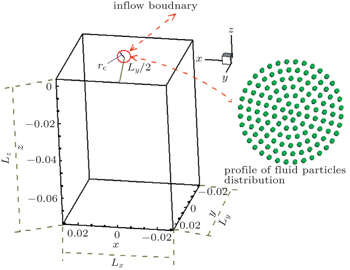Fig. 12. Sketch of 3D jet bulking.

Figure 13 illustrates the instability phenomenon of jet coiling and w-velocity contour, and the jet coiling phenomenon of the Newtonian fluid is also shown for comparison. The parameters are as follows: the jet width D = 5 mm(D = 2rc), Lx = Ly = 5 cm, Lz = 6 cm, U = − 1 m·s−1, Lz/D = 12, the reference density ρ0 = 1.1 × 103 k·gm−3, the gravitational acceleration gz = − 9.81 m·s−2, kinematic viscosity υ0 = 0.02 m2·s−1, speed of sound c = 8 m·s−1, β0 = 0.4, λ0b = 0.005 s (We = 1), λ0b = 0.5 s (We = 100), Re = 0.25, and the time-step Δt = 10−6 s.

According to previous research,[34,35] the phenomenon of jet coiling based on the Newtonian fluid will occur, if Re < 0.5 and Lz/D > 10 or Re < 1.2 and Lz/D > 7 are satisfied. From Fig. 13, we can see that the jet coiling indeed occurs for the Newtonian fluid with Re = 0.25 and Lz/D = 12, the reason can be explained as follows. On the one hand, the effect of the viscous force on flow is much bigger than that of inertia force after the fluid has touched the solid plate, thereby leading to the slow flow of the fluid. On the other hand, the fluid is continuously injected from the nozzle. The results of instability phenomenon of jet coiling based on the Oldroyd-B fluid basically are consistent with those obtained by FDM with MAC method in Refs.  and . Also, the absolute value of w-velocity near the front of jet fluid is bigger than that of the inlet velocity before the jet fluid reaches the bottom of rectangle container (t = 0.027 s). Thus the required time for Oldroyd-B fluid to arrive at the bottom of the rectangular container is shorter than that for Newtonian fluid, and the jet coiling phenomenon of the Oldroyd-B fluid lags behind that of Newtonian fluid due to the shear thinning behavior of the Oldroyd-B fluid, which reduces the viscosity when subjected to shear strain and is the unique characteristic of the non-Newtonian fluid. The w-velocity of the front of the jet fluid increases then decreases with time going by. Comparing Fig. 13 with the numerical results in Refs. , , and , we can obtain the conclusion that it is credible for the SPH_CS_SP method to simulate the 3D viscoelastic free surface flows.

 Figure Option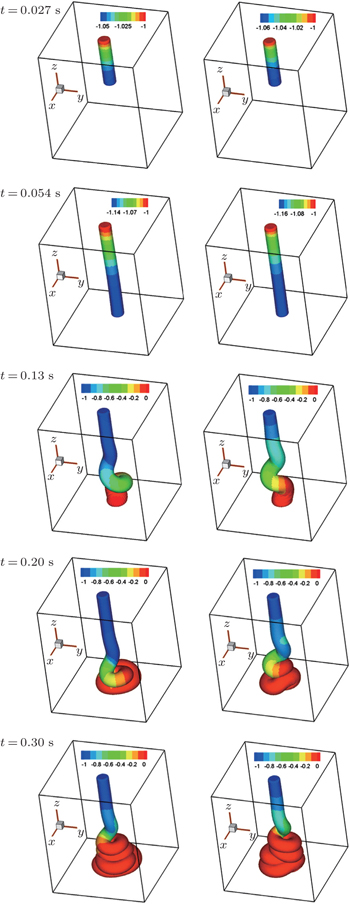Fig. 13. The flow and w-velocity contours of the jet bulking based on the Newtonian (first column) and Oldroyd-B (second column) fluid with Re = 0.25 and We = 1.

In order to clearly show the instability phenomenon of jet coiling, figure 14 displays the deformation processes of the center line of the jet based on the Newtonian fluid and Oldroyd-B fluid, which correspond to the results in Fig. 13 except for the results with We = 100. It can be seen that the jet coiling phenomenon indeed occurs which is more obvious than that in Fig. 13.

 Figure Option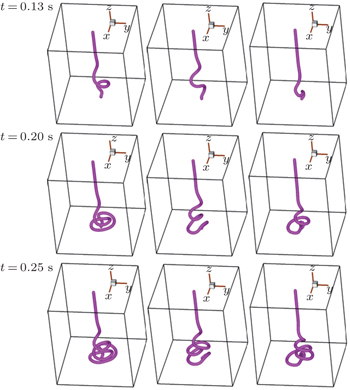Fig. 14. Deformation processes of the center line of jet with Re = 0.25: Newtonian fluid (first column); Oldroyd-B fluid with We = 1 (second column), and We = 100 (third column).

Next, the influences of macroscopic rheological parameters on the fluid deformation of filling process are predicted and discussed to further illustrate the complex phenomenon in the 3D viscoelastic filling process.

6.1. Influence of We

Observing Fig. 14, we can easily find that We has an important effect on the deformation process of viscoelastic jet fluid, and the phenomenon of jet coiling may occur for higher We at lower Reynolds number (We=100 in Fig. 14).

To further demonstrate the influence of We on the deformation process of jet coiling, we investigate the fluid deformations and the jet lengths of the jet buckling for the Oldroyd–B fluid with different Weissenberg numbers at Re=0.6. Here the jet length is defined as the distance from the jet front to the nozzle. The employed parameters are as follows: D = 6 mm, Lx = Ly = 6 cm, Lz = 5 cm (Lz/D = 8.3), U = −0.5 m·s−1, Δt = 5 × 10−6 s, υ0 = η/ρ0 = 0.005 m2·s−1 (Re = 0.6), λ0b = 0.006 s (We = 1), λ0b = 0.03s (We = 5), λ0b = 0.6s (We = 100), and the other parameters are the same as those in Fig. 13. The results are shown in Fig. 15.

 Figure Option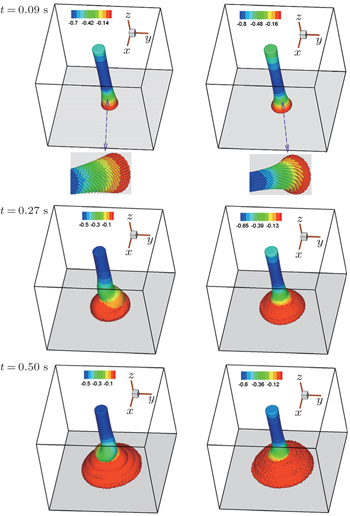Fig. 15. Filling deformation processes based on the Oldroyd-B fluid with Re = 0.6, We = 1 (first column) and 100 (second column) at different times.

From Fig. 15, we can find that the instability phenomenon of jet coiling appears with We=1 at t = 0.5s, but it does not occur when We changes from 1 to 100 due to the effect of elastic stress and shear thinning. The reason is that moreover, the forefront of the jet fluid is warped as the jet coiling happens, which may be obviously observed from Fig. 15 at the initial stage of jet fluid impacting on the container bottom (at t = 0.09 s). The warped distortion of jet fluid may be another reason for generating the instability phenomenon of jet coiling, which was not mentioned in previous studies.[30,34,35]

Figure 16 shows the variations of jet length of the jet bulking with time for different values of Weissenberg numbers. It can be observed that a longer jet length is achieved at a higher Weissenberg number. The reason is explained as follows. The increasing of We indicates that the relaxation time of the fluid increases, now the shear thinning character of the fluid instead of the elasticity dominates the flow in a short time before the fluid retracts. The conclusion is consistent with the remarks in Refs.  and .

 Figure Option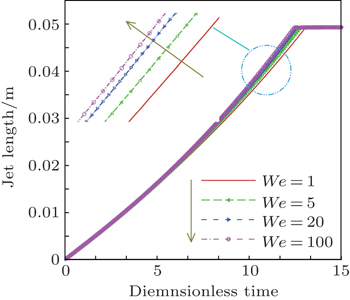Fig. 16. Variations of jet length of jet bulking with time for different values of We.
6.2. Influence of Re

Another macroscopic parameter which has an important influence on the deformation of jet fluid is the Reynolds number. Comparing Fig. 13 with Fig. 15, it is easy to find that the jet coiling phenomenon is weak as the Reynolds number increases.

In order to further show the effect of the Reynolds number on the viscoelastic flow, figure 17 displays the deformation processes of the jet buckling for the Oldroyd-B fluid with Re=1, 5, and 10. The parameters are as follows: D = 8 mm, Lx = Ly = 8 cm, Lz = 6 cm (Lz/D = 7.5), U = –0.5 m·s−1, λ0b = 0.006 s (We = 1), υ0 = 0.004 m2·s−1 (Re = 1), υ0 = 0.0008 m2·s−1 (Re = 5), υ0 = 0.0004 m2·s−1 (Re = 10), and the other parameters are the same as those in Fig. 15.

 Figure Option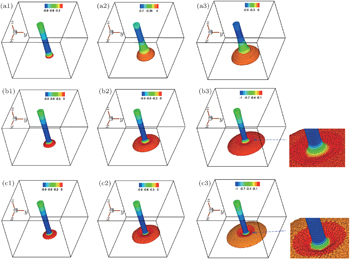Fig. 17. Deformation processes of jet buckling of the Oldroyd-B fluid at different times (t = 0.09 s, t = 0.21 s, t = 0.039 s) with different Reynolds numbers: Re = 1 ((a1)–(a3)), 5 ((b1)–(b3)), and 10 ((c1)–(c3)).

From Fig. 17, we can find that the filling processes are stable at Re=1 (Figs. 17(a1)17(a3)), which is required in the industrial manufacture. The phenomenon of jet coiling disappears as the Reynolds number continues to increase untilRe ≥ 1. As the Reynolds number increases up to 5 or 10, the concave phenomenon of forefront fluid appears as the jet fluid impacts on the container bottom (Figs. 17(b3)17(c3)). The more serious concave tendency is achieved as the Reynolds number increases (Figs. 17(b3)17(c3)). The concave phenomenon of jet fluid is unstable, which will generate some gas bubbles in the filling process.

7. Conclusions

In this work, the flow behavior of 3D jet buckling problem based on the Oldroyd-B model is simulated and predicted by a corrected particle scheme (SPH_CS_SP) method. The accuracy of the SPH_CS_SP method and the necessity of the shifting particle technique are first verified by two benchmark problems, that is, the Poiseuille flow and Taylor–Green flow, respectively. Then, the validity of the proposed SPH_CS_SP method to simulate the viscoelastic free surface is shown by the polymeric fluid flow around a periodic array of cylinders. Finally, the present method with MPI parallelization technique is further used to simulate the 3D jet coiling problem of filling process. The influences of macroscopic parameters on the instability phenomena are also investigated. Some conclusions can be drawn from the numerical results below.

Reference
 1 Owens R GPhillips T N2002Computational RheologyLondonImperial College Press1 2 Cleary P WMonaghan J J 1999 J. Comput. Phys. 148 227 3 Feng ZWang X DOuyang J 2013 Chin. Phys. B 22 074704 4 Chen F ZQiang H FGao W R 2014 Acta Phys. Sin. 63 130202 (in Chinese) 5 Gingold R AMonaghan J J 1997 Mon. Not. R. Astron. Soc. 181 375 6 Liu W KJun SZhang Y F 1995 Int. J. Numer. Method Fluids 20 1081 7 Li S FLiu W K 1999 Int. J. Numer. Method Eng. 45 251 8 Li S FLiu W K 1999 Int. J. Numer. Method Eng. 45 251 9 Morris J PFox P JZhu Y 1997 J. Comput. Phys. 136 214 10 Fang JParriaux ARentschler MAncey C 2009 Appl. Numer. Math. 59 251 11 Liu M BLiu G R 2010 Arch. Comput. Method Eng. 17 25 12 Hu X YAdams N A 2007 J. Comput. Phys. 227 264 13 Shao SLo EYM 2003 Adv. Water Resour. 26 787 14 Hu X YAdams N A 2006 J. Comput. Phys. 213 844 15 Ellero MTanner R I 2005 J. Non-Newtonian Fluid Mech. 132 61 16 Liu M BLiu G R 2006 Appl. Numer. Math. 56 19 17 Quinlan N JBasa MLastiwka M 2006 Int. J. Numer. Method Eng. 66 2064 18 Monaghan J J 2000 J. Comput. Phys. 159 290 19 Chen J KBeraun J E 2000 Comput. Methods Appl. Mech. Engrg. 190 225 20 Liu M BXie W PLiu G R 2005 Appl. Math. Model. 29 1252 21 Zhang G MBatra R C 2007 J. Comput. Phys. 222 374 22 Zhang G MBatra R C 2009 Comput. Mech. 43 321 23 Bonet JLok T S L 1999 Comput. Methods Appl. Mech. Engrg. 180 97 24 Oger GDoring MAlessandrini BFerrant P 2007 J. Comput. Phys. 225 1472 25 Shadloo M SZainali AYildiz MSuleman A 2012 Int. J. Numer. Method Eng. 89 939 26 Basa MQuinlan N JLastiwka M 2009 Int. J. Numer. Method Fluids 60 1127 27 Ren J LOuyang JJiang TLi Q 2012 Comput. Mech. 49 643 28 Xu RStansby PLaurence D 2009 J. Comput. Phys. 228 6703 29 Wendland H 1995 Adv. Comput. Math. 4 389 30 Tomé M FCastelo AFerreira V GMcKee S 2008 J. Non-Newtonian Fluid Mech. 154 179 31 Liu A WBornside D EArmstrong R CBrown R 1998 J. Non-Newtonian Fluid Mech. 77 153 32 Ellero MAdams N 2011 Int. J. Numer. Method Eng. 86 1027 33 Vazquez-Quesada AEllero M 2012 J. Non-Newtonian Fluid Mech. 167�?68 1 34 Tomé M FGrossi LCastelo ACuminato J AMangiavacchi NFerreira V Gde Sousa F SMcKee S 2004 J. Non-Newtoian Fluid Mech. 123 85 35 Oishi C MTomé M FCuminato J AMcKee S 2008 J. Non-Newtoian Fluid Mech. 227 7446